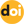The peer-reviewed scientific and technology journal. ISSN: 0204-3327

# Assessment of critical condition for IBR reactor with subcritical block in the equilibrium modehttps://doi.org/10.26583/npe.2017.1.08

### UDC: 621.039.5

A system consisting of a fast batch pulsed reactor of the IBR-type and subcritical block (thermal in terms of neutronics) is examined. The reactor is equipped with a reactivity modulator, which provides for a short time of the «transition» of the system from deeply subcritical state to supercritical state on prompt neutrons and back. During the intervals between pulses the system is in the deeply subcritical state.

This type of reactor system can function in the equilibrium (static) mode in the case when the critical condition for the kinetic parameters describing its operation is fulfilled. Description of neutron kinetics is made in two-point approximation. It is assumed here that the change in reactivity occurs periodically according to parabolic law. Numerical simulation of the critical condition is extremely difficult and time-consuming, while analytical representation is almost impossible due to the necessity to solve ordinary differential equations with variable coefficients.

Methodology is suggested in the present paper allowing approximating the parameters of the coupled system consisting of the batch pulsed reactor and subcritical block operating in the equilibrium mode. Analytical relations in a quadrature form for the calculation of «critical» condition of such a system in «frequent» pulses approximation, when one can neglect the collapse of the delayed neutron precursors during the interval between pulses, are obtained.

Calculations of the critical condition are illustrated using the example of a laser system consisting of a batch pulsed reactor IBR-2 and subcritical block multiplying neutrons, in which fission energy is converted into laser emission energy.

Estimates of critical system parameters were obtained using analytical expressions, as well as by direct numerical calculations using STIK code modeling the kinetics of neutrons in the system under examination in the two-point approximation.

Good agreement between the results of direct calculations and estimations obtained using analytical expressions was demonstrated.

1. Shabalin E.P. Impulsnye reaktory na bystrykh nejtronakh [Pulsed fast neutron reactors]. Moscow. Atomizdat Publ., 1976, 248 p. (in Russian).
2. Bondarenko I.I., Stavisskij Yu.Ya.Impul’snyj rezhim raboty bystrogo reaktora [The pulsed mode of operation of the fast reactor]. Atomnaya energiya, 1959, v. 7, iss. 5, p. 417 (in Russian).
3. Barzilov A.P., Gulevich A.V., D’yachenko P.P., Zrodnikov A.V., Kononov V.N., Kukharchuk O.F., Pupko V.YA. Lazernaya sistema impul’sno-periodicheskogo dejstviya s nakachkoj ot yadernogo reaktora tipa IBR [The laser system with a pulsed-periodic action pumped from the nuclear reactor of IBR- type]. Proc. of the Int. Conf. «Physics of nuclear-excited plasma and problems of lasers with nuclear pumping (LYaN’94)». Sarov (Arzamas-16). RFYaC-VNIIEF Publ., 1994, v. 2, p. 186 (in Russian).
4. Dyachenko P.P., Zrodnikov A.V., Prokhorov A.M. Korobkin V.V., Poupko V.Ya. Concept of a Combined ICF Power Plant and a Fission Reactor-Laser Driver. Fusion Techn., 1991, v. 20, no. 4.
5. Kolesov V.F., Petrov Yu.V., Shtaraev S.K. Kinetika sistemy svyazannykh impul’snykh reaktorov.[Kinetics of the system of coupled pulsed reactors].Atomnaya energiya, 1975, v. 39, iss. 6, p. 392 (in Russian).
6. Gulevich A.V., Dyachenko P.P., Zrodnikov A.V., Kukharchuk O.F. Svyazannye reaktornye sistemy impulsnogo deystviya [Pulsed Coupled Reactor System]. Moscow. Energoatomizdat Publ., 2003, 360 p. (in Russian).
7. Bell D., Glasstone S. Teoriya yadernykh reaktorov [Nuclear Reactor Theory]. Moscow. Atomizdat Publ., 1974, 493 p. (in Russian).
8. Komata M. On the Derivation of Avery’s Coupled Reactor Kinetics Equations. Nucl. Sci. and Eng., 1968, v. 38, p. 193.
9. Stevenson M., Gage S. Application of a Coupled Fission Mode Approach to Modular Reactor Kinetics. J. of Nucl. Ener., 1970, v. 24, no. 1, p. 1.
10. Thayer G., Miley G., Jones B. Experimental Studies of Large Amplitude Transients in Weakly Coupled Cores. Trans. of Amer. Nucl. Soc., 1972, v. 15, no. 2, p. 925.
11. Thayer G., Miley G., Jones B. An Experimental Study of Two Coupled Reactors. Nucl. Techn., 1975, v. 25, no. 1, р. 56.
12. Difilippo F., Waldman R. The Kinetics of a Coupled Two-Core Nuclear Reactor. Nucl. Sci. Eng., 1976, v. 61, no. 1, p. 60.
13. Takezawa H., Obara T., Gulevich A., Kukharchuk O. Criticality Analysis of Pulse Core and Laser Module Coupled Small Reactor with Low Enriched Uranium. Progress in Nuclear Energy, 2008, v. 50, no. 2-6, p. 304.
14. Kamke EH. Spravochnik po obyknovennym differencial’nym uravneniyam [Handbook on ordinary differential equations.]. Moscow. Nauka Publ., 1971. 589 p. (in Russian).
15. Gulevich A.V., Kachanov B.V., Kuharchuk O.F. Modeli i programmy rascheta dinamicheskikh kharakteristik reaktorno-lazernykh sistem [Models and codes for calculation of dynamic characteristics of the reactor-laser systems]. Preprint FEI-2454, Obninsk, 1995, 23 p. (in Russian).